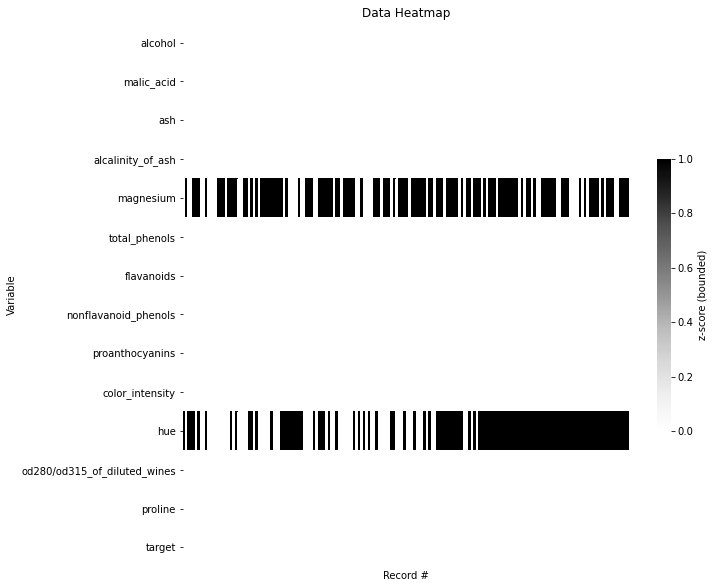# Data Heatmap¶

:

import pandas as pd
from data_describe import data_heatmap

:

from sklearn.datasets import load_wine
df = pd.DataFrame(data.data, columns=list(data.feature_names))
df['target'] = data.target


## Basic heatmap¶

:

data_heatmap(df):

Heatmap Widget showing standardized values.


## Missing values only¶

:

# Create missing values
df['hue'] = df['hue'].map(lambda x: None if x < 1.05 else x)
df['magnesium'] = df['magnesium'].map(lambda x: None if x%2 ==0 else x)

:

data_heatmap(df, missing=True):

Heatmap Widget showing missing values.


## Interactive (Plotly)¶

:

data_heatmap(df, viz_backend="plotly")

C:\workspace\data-describe\data_describe\compat\_notebook.py:32: JupyterPlotlyWarning:

Are you running in Jupyter Lab? The extension "jupyterlab-plotly" was not found and is required for Plotly visualizations in Jupyter Lab.


None

:

Heatmap Widget showing standardized values.

:

data_heatmap(df, viz_backend="plotly", missing=True)

None

:

Heatmap Widget showing missing values.